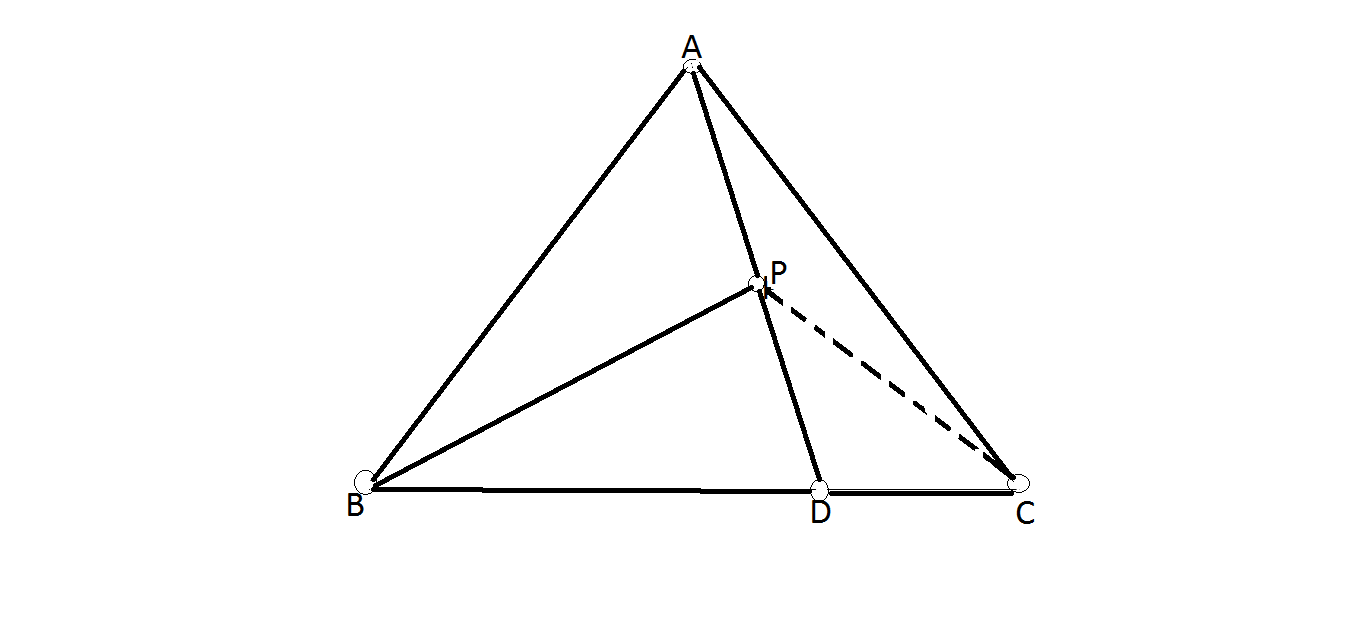# Find the angle

Geometry Level 4Let $D$ be the point on the base $BC$ of an isosceles triangle $ABC$ with $AB=AC$ and $m(BAC)=80^\circ$, such that $BD : DC = 2 : 1$, and let $P$ be the point on the segment $AD$ such that $m(BAC) = m(BPD)$. Find $m(DPC)$ in degrees.

×

Problem Loading...

Note Loading...

Set Loading...